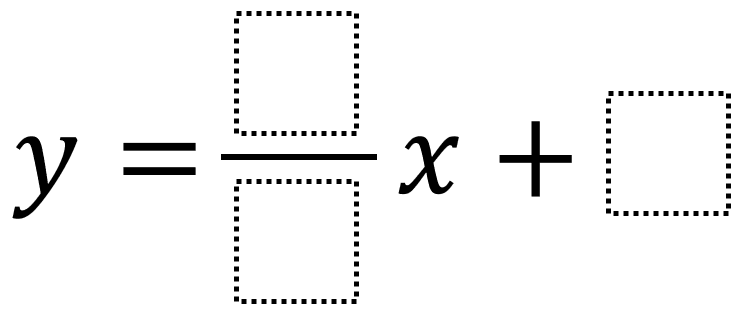# Graphing Linear Equations 1

Directions: Using the integers -9 to 9 at most one time each, fill in the boxes to make two linear equations which go through (5, 4): one with a negative slope and one with a positive slope. You may reuse all the integers for each equation.### Hint

How can you determine whether your line intersects the point (5, 4) algebraically? How can you determine whether your line intersects the point (5, 4) graphically? Here’s a link to an already set up Desmos version.